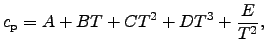# 3.4 Heat Capacitance

The heat capacitance is modeled according to a commonly used empirical formula introduced in [196,197](3.21)

where appropriate coefficients for this equation exist in tabular form and in diagrams, see for instance [197,198,199,131,132,133,134,135,136,137,138] and Figure 2.9. Equation (3.21) yields very good results in simulations when compared to measurements. However, these coefficients are valid for the specified temperature range only.